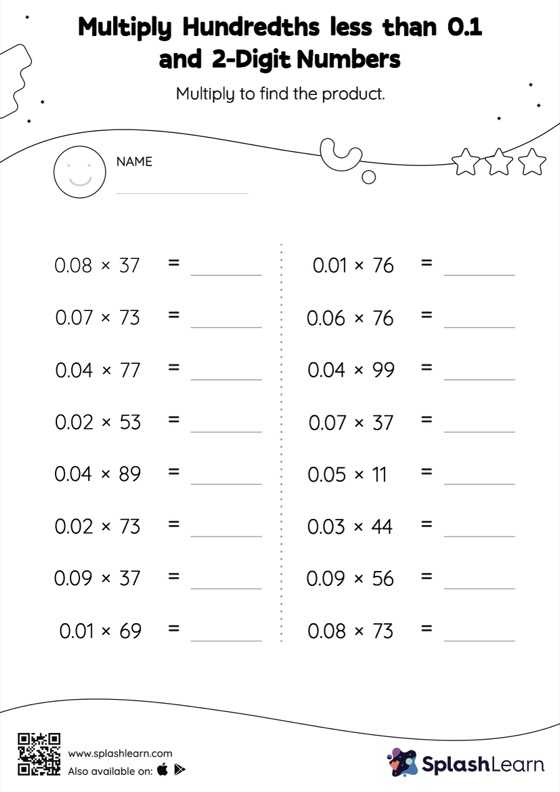# Multiply Hundredths less than 0.1 and 2-Digit Numbers: Horizontal Multiplication Worksheet

Home > Multiply Hundredths less than 0.1 and 2-Digit Numbers: Horizontal MultiplicationMultiply hundredths less than 0.1 And 2-digit numbers worksheet is a great way to help learners become fluent with the concept of multiplication. When multiplying a decimal by a two-digit number, students can omit the decimal point and add it when they write the answer. Learners have numerous opportunities to put this idea into practice with this multiply hundredths less than 0.1 And 2-digit numbers worksheet. In this worksheet, students practice solving problems written in the horizontal format. How numbers are laid out in a problem affects the method a student employs to solve it. Therefore to develop actual fluency and mastery of multiple strategies, students must practice different formats.